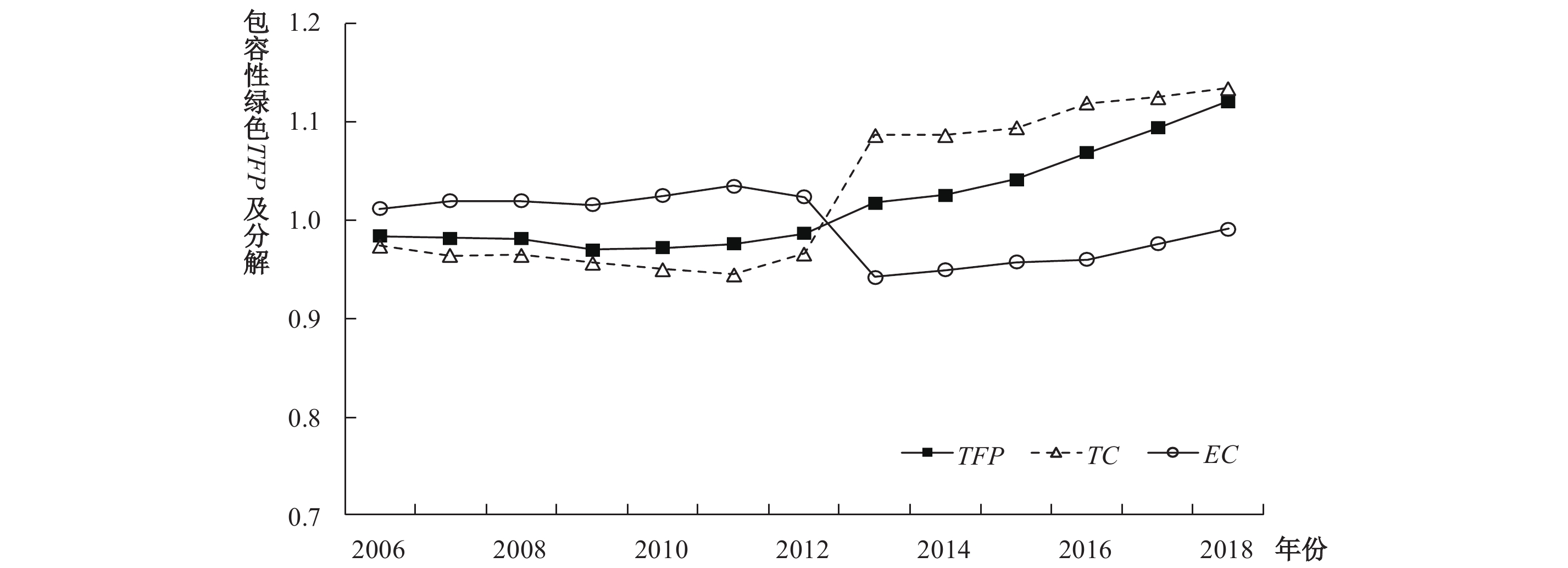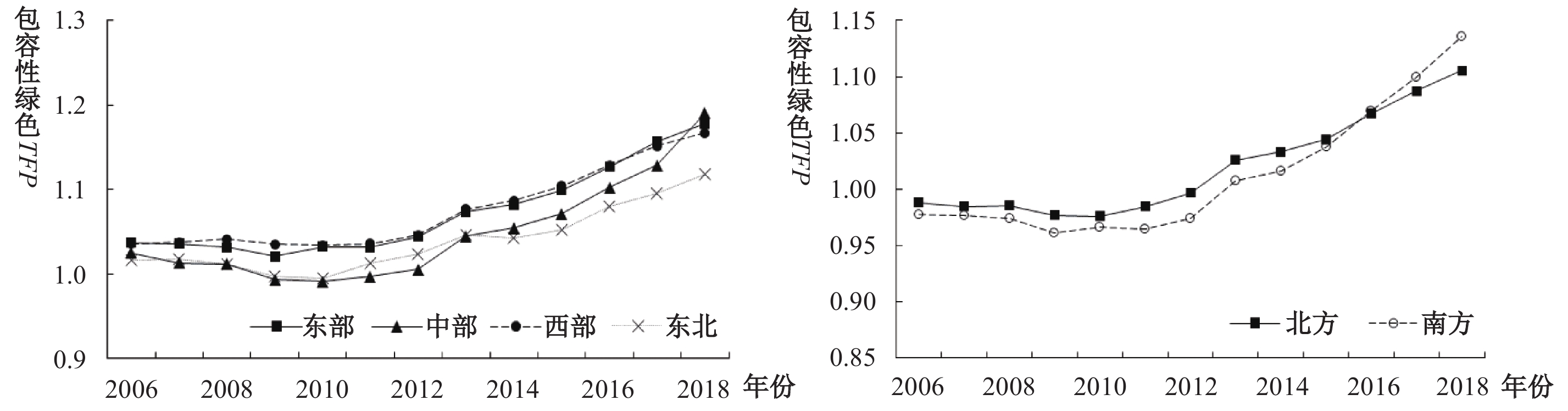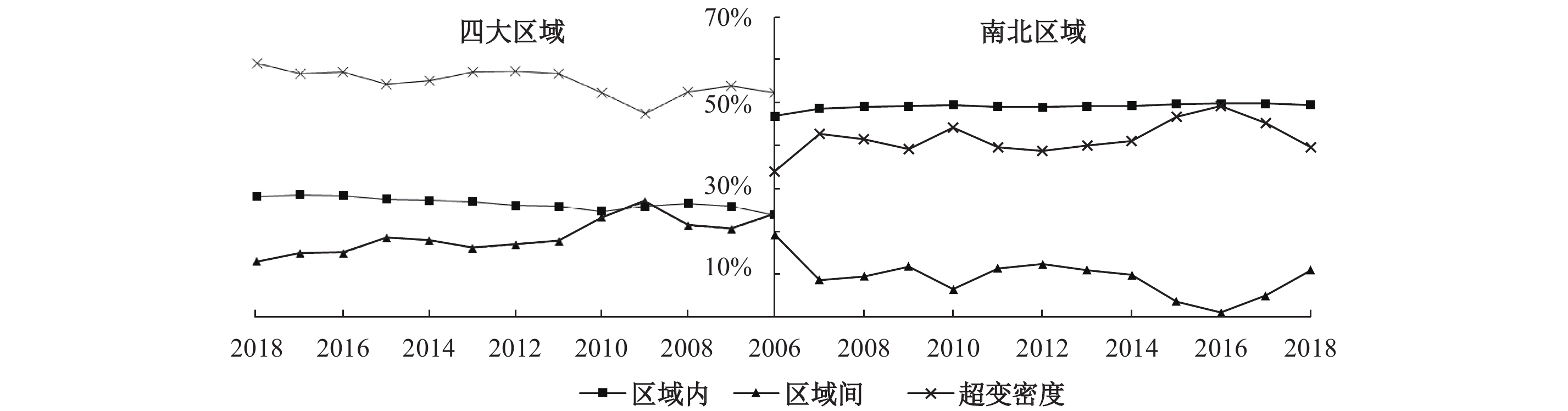﻿ 中国经济高质量发展水平及差异探源Li Hua, Dong Yanling. China’s high-quality economic development level and the source of differences: based on the inclusive green tfp perspective[J]. Journal of Finance and Economics, 2021, 47(8): 4-18.China’s High-quality Economic Development Level and the Source of Differences: Based on the Inclusive Green TFP Perspective
Li Hua, Dong YanlingSchool of Economics，Shandong University，Jinan 250100，China
Key words: regional differences    structural decomposition    the inclusive green TFP    high-quality economic development

(一) 理论模型与指标体系构建

1. 经济高质量发展的内涵。准确理解经济高质量发展的内涵是对其进行科学评估的重要前提。目前，评价方式主要涉及全要素生产率测度和指标体系构建两大类。由于前者可聚焦经济运行效能，有助于把握经济发展方式的转变，因此本文从全要素生产率视角对经济高质量发展进行水平测度及差异探源。作为经济增长的动力源泉，全要素生产率是技术进步的重要衡量指标，其本身就是“创新”的体现。“绿色”增长中，能源和环境既是经济增长的内生因素，也是质量提高的刚性约束。“包容性”增长则强调广大群众要共享经济增长的福利，缩小城乡和区域居民收入消费差距，落实“共享”“协调”发展理念。然而，传统全要素生产率未将环境和社会因素纳入其中，难以对经济高质量发展的绩效进行较为全面的科学评估。在以国内大循环为主体的新发展格局背景下，包容性绿色全要素生产率综合体现了创新、协调、绿色、共享理念，能够较好地衡量经济高质量发展水平。

2. 理论模型：包容性绿色索洛模型。全要素生产率的测度主要源于索洛模型。由于经典的索洛模型仅考虑资本和劳动作为投入的情况，获得的全要素生产率实质上是技术进步。本文借鉴王兵和刘光天（2015）的研究，同时考虑环境、社会和效率因素，构建了更能反映现实情况的包容性绿色索洛模型：

 $Y - {C_1} - {C_2} = \left( {A\theta } \right)\left( {{L^\alpha }{K^\beta }{E^\gamma }} \right)$ (1)

 $A\theta = {{\left( {Y - {C_1} - {C_2}} \right)} / {\left( {{L^\alpha }{K^\beta }{E^\gamma }} \right)}}$ (2)

 $TFP = \frac{{\Delta A}}{A} + \frac{{\Delta \theta }}{\theta } = \frac{{\Delta \left( {Y - {C_1} - {C_2}} \right)}}{{Y - {C_1} - {C_2}}} - \alpha \frac{{\Delta L}}{L} - \beta \frac{{\Delta K}}{K} - \gamma \frac{{\Delta E}}{E}$ (3)

3. 指标体系的构建。基于经济高质量发展内涵，本文借鉴王兵等（2010）、聂长飞和简新华（2020）、李华和董艳玲（2020）等文献，构建了中国包容性绿色全要素生产率的指标测度体系，具体见表1。对于非期望产出，本文不但考虑传统污染物，还将近年来引发关注的雾霾也纳入其中，以综合反映经济增长中的环境污染情况；考虑到不平等问题主要分为收入和消费两个方面（周龙飞和张军，2019），如只考虑一个角度，则存在一定局限性（邹红等，2013），因此本文基于城乡和区域视角，从居民收入和消费支出层面选取衡量社会不公的4个代表性指标。

 一级 二级 三级 衡量变量 投入 投入要素 资本投入 资本存量（详见刘华军等（2018），其中折旧率参考吴延瑞（2008）的研究） 劳动投入 从业人员 能源投入 能源消费量 产出 期望产出 地区生产总值 实际GDP 非期望产出—环境污染 二氧化硫排放量 包括工业和生活 废水排放量 包括工业和生活 工业固体废物 工业排放 烟尘排放量 包括工业和生活 PM2.5 年均浓度（微克/立方米） 非期望产出—社会不公 城乡居民收入差距 城镇居民可支配收入/农村居民可支配收入 城乡居民消费差距 城镇居民消费支出/农村居民消费支出 区域居民收入差距 （各省城镇居民可支配收入−全国均值）绝对值/全国均值 区域居民消费差距 （各省城镇居民消费支出−全国均值）绝对值/全国均值

(二) 分析方法

1. 包容性绿色全要素生产率水平的测度。本文借鉴陈红蕾和覃伟芳（2014）、王谦和董艳玲（2018）、陈明华等（2020a）的做法，将

$X=\left({x}_{1},{x}_{2}\cdots {x}_{L}\right)\in {R}_{+}^{m}$

${Y^g} = \left( {y_1^g,y_2^g \cdots y_L^g} \right) \in R_ + ^{{s_1}}$

${Y^b} = \left( {y_1^b,y_2^b \cdots y_L^b} \right) \in R_ + ^{{s_2}}$

 $PPS=\left\{ \left. \left( \bar{x},{{{\bar{y}}}^{g}},{{{\bar{y}}}^{b}} \right) \right|\bar{x}\ge \sum\limits_{\begin{matrix} j=1 \\ j\ne 0 \\ \end{matrix}}^{\text{L}}{{{\lambda }_{j}}{{x}_{j}}},{{{\bar{y}}}^{g}}\le \sum\limits_{\begin{matrix} j=1 \\ j\ne 0 \\ \end{matrix}}^{\text{L}}{{{\lambda }_{j}}{{x}_{j}}},{{{\bar{y}}}^{g}}{{\lambda }_{j}}y_{j}^{g},{{{\bar{y}}}^{b}}\ge \sum\limits_{\begin{matrix} j=1 \\ j\ne 0 \\ \end{matrix}}^{\text{L}}{{{\lambda }_{j}}{{x}_{j}}},{{{\bar{y}}}^{g}}{{\lambda }_{j}}y_{j}^{b},l\le e\lambda \le u,{{\lambda }_{j}}\ge 0 \right\}$ (4)

$\lambda =\left({\lambda }_{1},{\lambda }_{2}\cdots {\lambda }_{L}\right)$
L维权重向量；lu衡量规模报酬假设，有不变和可变两种，符号依次为
$\left(l=0,u=\infty \right)$
$\left(l=1,u=1\right)$
；向量
${s^ - } \in R_ + ^m$
${s^b} \in R_ + ^{{s_2}}$

${s^g} \in R_ + ^{{s_1}}$

 $ML{I_g}\left( {{x^{t + 1}},{y^{gt + 1}},{y^{bt + 1}},{x^t},{y^{gt}},{y^{bt}}} \right) = \frac{{{E^g}\left( {{x^{t + 1}},{y^{gt + 1}},{y^{bt + 1}}} \right)}}{{{E^g}\left( {{x^t},{y^{gt}},{y^{bt}}} \right)}}$ (5)

MLI测算了DMUt期到t+1期包容性绿色全要素生产率的变动。若MLIg=1，则说明没有变化；若MLIg>1，则说明有所提高；若MLIg<1，则说明有所降低。MLI根据内部构成可分为效率变化（EC）和技术进步（TC），即MLI=EC×TC，其中：

 $EC = \frac{{{E^{t + 1}}\left( {{x^{t + 1}},{y^{gt + 1}},{y^{bt + 1}}} \right)}}{{{E^t}\left( {{x^t},{y^{gt}},{y^{bt}}} \right)}}$ (6)
 $TC = \frac{{{E^g}\left( {{x^{t + 1}},{y^{gt + 1}},{y^{bt + 1}}} \right)}}{{{E^{t + 1}}\left( {{x^{t + 1}},{y^{gt + 1}},{y^{bt + 1}}} \right)}}\frac{{{E^t}\left( {{x^t},{y^{gt}},{y^{bt}}} \right)}}{{{E^g}\left( {{x^t},{y^{gt}},{y^{bt}}} \right)}}$ (7)

2. 包容性绿色全要素生产率的空间差异测度及来源。本文运用Dagum基尼系数对我国包容性绿色全要素生产率的空间差异进行刻画。式（8）呈现了基尼系数G的含义（Dagum，1997）。式中，yjiyhr）是第jh）个区域任一省市包容性绿色全要素生产率的代理符号，n是省市数量代理符号，μ表示四大区域（或南北区域）30个省市包容性绿色全要素生产率的均值，k表示区域数量，从而得到我国包容性绿色全要素生产率的总体差异。

 $G = \frac{{\displaystyle\sum\limits_{j = 1}^k {\sum\limits_{h = 1}^k {\sum\limits_{i = 1}^{{n_j}} {\sum\limits_{r = 1}^{{n_h}} {\left| {{y_{ji}} - {y_{hr}}} \right|} } } } }}{{2{n^2}\mu }}$ (8)
 $G = {G_w} + {G_{nb}} + {G_t}$ (9)

3. 包容性绿色全要素生产率差异的结构来源。借鉴刘华军等（2018）的研究，本文基于结构组成视角，通过方差分解方法，测度技术效率差异和技术进步差异对包容性绿色全要素生产率差异的贡献程度。具体公式如下：

 $MLI = EC \times TC$ (10)

 $\ln \left( {MLI} \right) = \ln \left( {EC} \right) + \ln \left( {TC} \right)$ (11)

 $\operatorname{var} \left( {\ln MLI} \right) = \operatorname{cov} \left( {\ln MLI,\ln EC} \right) + \operatorname{cov} \left( {\ln MLI,\ln TC} \right)$ (12)

 $1 = \frac{{\operatorname{cov} \left( {\ln MLI,\ln EC} \right)}}{{\operatorname{var} \left( {\ln MLI} \right)}} + \frac{{\operatorname{cov} \left( {\ln MLI,\ln TC} \right)}}{{\operatorname{var} \left( {\ln MLI} \right)}}$ (13)

(一) 总体水平与来源分析图 1 包容性绿色TFP及分解的演变态势

(二) 区域层面图 2 四大区域和南北区域包容性绿色TFP的变化趋势图 3 四大区域和南北区域包容性绿色TFP分解指标的演变情况

(三) 省际层面图 4 各省市包容性绿色TFP均值排名情况

 类型 东部 中部 西部 东北 北方 南方 明星型 北京、浙江、 山西 重庆、内蒙古、四川 辽宁 北京、内蒙古、 上海、重庆、 上海、广东、 辽宁、山西、 广东、浙江、 山东 山东 四川 平庸型 河北、江苏 湖北、安徽、江西 宁夏、青海、 吉林 宁夏、吉林、 江苏、江西、 甘肃、贵州、 甘肃、青海、 贵州、安徽、 陕西、云南 陕西、河北 云南、湖北 落后型 海南、天津、福建 河南、湖南 新疆、广西 黑龙江 新疆、河南、天津、黑龙江 湖南、海南、广西、福建图 5 各省市包容性绿色TFP分解指标对比情况

(一) 地区差异来源图 6 中国包容性绿色TFP空间差异来源的变化趋势 注：纵坐标表示差异来源的贡献率，横坐标表示年份。

(二) 结构差异来源

 年份 2006 2007 2008 2009 2010 2011 2012 TC贡献 85.82 73.29 66.21 73.67 75.91 73.83 78.85 EC贡献 14.18 26.71 33.79 26.33 24.09 26.17 21.15 年份 2013 2014 2015 2016 2017 2018 均值 TC贡献 67.39 69.16 66.14 67.55 72.48 67.09 72.11 EC贡献 32.61 30.84 33.86 32.45 27.52 32.91 27.89图 7 四大区域和南北区域包容性绿色TFP差异结构分解的变化趋势

① 考虑到DEA模型进行效率测度时会受到投入产出指标数量的限制，本文使用简单加权平均法将环境污染下的子指标拟合为一个综合指标，社会不公也采取同样处理方式。

② 区域层面旨在衡量不同省市与全国范围内居民可支配收入（或消费）的差异程度。考虑到2013年前后农村居民可支配收入出现统计口径和指标名称变化，为了降低对测度结果的影响，区域层面采用城镇居民作为代表来进行研究。

③ 本文没有将西藏、香港、澳门和台湾地区作为研究对象。

④ 主要数据来源包括各省市统计年鉴、《中国统计年鉴》、国家统计局、华盛顿大学Atmospheric Composition Analysis Group官方网站以及《中国能源统计年鉴》等。

⑤ 根据国家统计局2011年6月13日相关文件，我国四大区域划分情况如下：东部包括北京、天津、河北、山东、江苏、浙江、上海、福建、广东、海南10个省份；中部包括山西、安徽、江西、河南、湖北、湖南6个省份；西部包括内蒙古、广西、重庆、四川、贵州、云南、陕西、甘肃、青海、宁夏、新疆11个省份；东北则包括辽宁、吉林、黑龙江3个省份。本文以秦岭—淮河作为南北地区分界线，北方包括北京、天津、河北、山西、内蒙古、辽宁、吉林、黑龙江、山东、河南、陕西、甘肃、青海、宁夏、新疆15个省份，南方包括上海、江苏、浙江、安徽、福建、江西、湖北、湖南、广东、广西、海南、重庆、四川、贵州、云南15个省份。

⑥ 因篇幅所限，模型的公式不一一列出，详见王谦和董艳玲（2018）。

⑦ 全局参比Malmquist模型是由Pastor和Lovell（2005）提出的一种Malmquist指数计算方法，以所有时期作为参考集，各期参考的是共同前沿。

Dagum 基尼系数分解思路如下：（1）计算四大区域30个省市的包容性绿色TFP以及全国的基尼系数；（2）计算各区域包容性绿色TFP的基尼系数，进而得到区域内差异对全国总差异的贡献；（3）按照四大区域包容性绿色TFP均值对区域降序排序，区域内部各省市也降序排序，计算此情况下区域间差异对总差异的贡献；（4）按照30个省市包容性绿色TFP均值降序排序，并与按照区域均值降序排序的数列对比，若出现个别省市离开所在区域升序或降序到其他区域而造成不同区域间交叉重叠的现象就是超变密度。因此，超变密度反映个别省市出现的“不合群”现象，若水平过高，进入高水平行列；相反，则退到低水平群体。

⑨ 因篇幅所限，相关的计算公式不一一列出，详见Dagum（1997）。

⑩ 借鉴邱斌等（2008）和陈超凡（2016）的调整方法，得到以2005年为基期的包容性绿色全要素生产率。

⑪ 内蒙古的包容性绿色全要素生产率水平较高主要得益于劳动投入相对较低，社会不公产出较少。在其他条件相对不变情况下，投入要素越少，非期望产出越低，效率就越高。

⑫ 福建的包容性绿色全要素生产率水平较低主要是由资本和劳动投入较多、社会不公产出较高造成的。

⑬ 限于篇幅，本文未列出中国包容性绿色TFP的总体差异数值；如有需要，可向作者索取。

  陈超凡. 中国工业绿色全要素生产率及其影响因素——基于ML生产率指数及动态面板模型的实证研究[J]. 统计研究, 2016(3): 53–62. DOI:10.3969/j.issn.1002-4565.2016.03.007  陈红蕾, 覃伟芳. 中国经济的包容性增长: 基于包容性全要素生产率视角的解释[J]. 中国工业经济, 2014(1): 18–30.  陈景华, 陈姚, 陈敏敏. 中国经济高质量发展水平、区域差异及分布动态演进[J]. 数量经济技术经济研究, 2020(12): 108–126.  陈明华, 张晓萌, 刘玉鑫, 等. 绿色TFP增长的动态演进及趋势预测——基于中国五大城市群的实证研究[J]. 南开经济研究, 2020a(1): 20–44.  陈明华, 刘玉鑫, 刘文斐, 等. 中国城市民生发展的区域差异测度、来源分解与形成机理[J]. 统计研究, 2020b(5): 54–67.  邓忠奇, 高廷帆, 朱峰. 地区差距与供给侧结构性改革——“三期叠加”下的内生增长[J]. 经济研究, 2020(10): 22–37.  李华, 董艳玲. 基本公共服务均等化是否缩小了经济增长质量的地区差距?[J]. 数量经济技术经济研究, 2020(7): 48–70.  李苏, 尹海涛. 我国各省份绿色经济发展指数测度与时空特征分析——基于包容性绿色增长视角[J]. 生态经济, 2020(9): 44–53.  刘长庚, 田龙鹏, 陈彬. 经济制度变迁、包容性增长与收入分配[J]. 财经科学, 2016(1): 78–87. DOI:10.3969/j.issn.1000-8306.2016.01.008  刘华军, 李超, 彭莹. 中国绿色全要素生产率的地区差距及区域协同提升研究[J]. 中国人口科学, 2018(4): 30–41.  刘帅. 中国经济增长质量的地区差异与随机收敛[J]. 数量经济技术经济研究, 2019(9): 24–41.  聂长飞, 简新华. 中国高质量发展的测度及省际现状的分析比较[J]. 数量经济技术经济研究, 2020(2): 26–47.  邱斌, 杨帅, 辛培江. FDI技术溢出渠道与中国制造业生产率增长研究: 基于面板数据的分析[J]. 世界经济, 2008(8): 20–31. DOI:10.3969/j.issn.1002-9621.2008.08.002  史修松, 赵曙东. 中国经济增长的地区差异及其收敛机制（1978~2009年）[J]. 数量经济技术经济研究, 2011(1): 51–62.  王兵, 刘光天. 节能减排与中国绿色经济增长——基于全要素生产率的视角[J]. 中国工业经济, 2015(5): 57–69.  王兵, 吴延瑞, 颜鹏飞. 中国区域环境效率与环境全要素生产率增长[J]. 经济研究, 2010(5): 95–109.  王谦, 董艳玲. 公共风险约束下中国地方财政支出效率评价与影响因素分析[J]. 财政研究, 2018(11): 46–61.  王一鸣. 百年大变局、高质量发展与构建新发展格局[J]. 管理世界, 2020(12): 1–12. DOI:10.3969/j.issn.1002-5502.2020.12.002  王宇昕, 余兴厚, 黄玲. 长江经济带包容性绿色增长的测度与区域差异分析[J]. 贵州财经大学学报, 2019(3): 89–98. DOI:10.3969/j.issn.1003-6636.2019.03.010  魏后凯, 年猛, 李玏. “十四五”时期中国区域发展战略与政策[J]. 中国工业经济, 2020(5): 5–22.  魏敏, 李书昊. 新时代中国经济高质量发展水平的测度研究[J]. 数量经济技术经济研究, 2018(11): 3–20.  吴武林, 周小亮. 中国包容性绿色增长测算评价与影响因素研究[J]. 社会科学研究, 2018(1): 27–37. DOI:10.3969/j.issn.1000-4769.2018.01.004  吴武林, 周小亮. 中国包容性绿色增长绩效评价体系的构建及应用[J]. 中国管理科学, 2019(9): 183–194.  吴延瑞. 生产率对中国经济增长的贡献: 新的估计[J]. 经济学（季刊）, 2008(3): 827–842.  杨汝岱, 朱诗娥. 公平与效率不可兼得吗?——基于居民边际消费倾向的研究[J]. 经济研究, 2007(12): 46–58.  杨雪星. 包容性绿色经济增长指数构建与实证研究——基于G20国家数据[J]. 福建论坛·人文社会科学版, 2014(6): 42–48.  张晓颖. 经济、环境、社会发展与人: 从可持续发展观到包容性绿色增长[J]. 江淮论坛, 2014(6): 93–98. DOI:10.3969/j.issn.1001-862X.2014.06.016  张勋, 万广华. 中国的农村基础设施促进了包容性增长吗?[J]. 经济研究, 2016(10): 82–96.  周龙飞, 张军. 中国城镇家庭消费不平等的演变趋势及地区差异[J]. 财贸经济, 2019(5): 143–160. DOI:10.3969/j.issn.1002-8102.2019.05.010  周小亮, 吴武林, 廖达颖. 我国区域包容性绿色增长测度与差异研究[J]. 科技进步与对策, 2018(6): 42–49.  周小亮, 吴武林. 中国包容性绿色增长的测度及分析[J]. 数量经济技术经济研究, 2018(8): 3–20.  周小亮. 包容性绿色发展: 理论阐释与制度支撑体系[J]. 学术月刊, 2020(11): 41–54.  邹红, 李奥蕾, 喻开志. 消费不平等的度量、出生组分解和形成机制——兼与收入不平等比较[J]. 经济学（季刊）, 2013(4): 1231–1254.  Albagoury S. Inclusive green growth in Africa: Ethiopia case study[R]. MPRA Working Paper No.74364, 2016.  Bouma J, Berkhout E. Inclusive green growth[R]. PBL Netherlands Environmental Assessment Agency, No.1708, 2015.  Dagum C. A new approach to the decomposition of the Gini income inequality ratio[J]. Empirical Economics, 1997, 22(4): 515–531. DOI:10.1007/BF01205777  Kessler J J, Slingerland S. Study on public private partnerships for contribution to inclusive green growth[R]. PBL Netherlands Environmental Assessment Agency, No.2557, 2015.  Khoday K, Perch L. China and the world: South-South cooperation for inclusive green growth[R]. Working Paper No.95, 2012.  Kumar S. Engendering liveable low-carbon smart cities in ASEAN as an inclusive green growth model and opportuni- ties for regional cooperation[R]. ERIA Working Papers No.DP-2015-57, 2015.  Pastor J T, Lovell C A K. A global Malmquist productivity index[J]. Economics Letters, 2005, 88(2): 266–271. DOI:10.1016/j.econlet.2005.02.013  World Bank. Inclusive green growth: The pathway to sustainable development[M]. Washington: World Bank Publication, 2012.